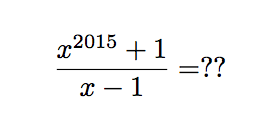# Remainder of polynomial divisionWhat is the remainder of the division of $x^{2015}+1$ by $x-1$?

Hint : This should take you no longer than 5 seconds to figure out the answer!

×